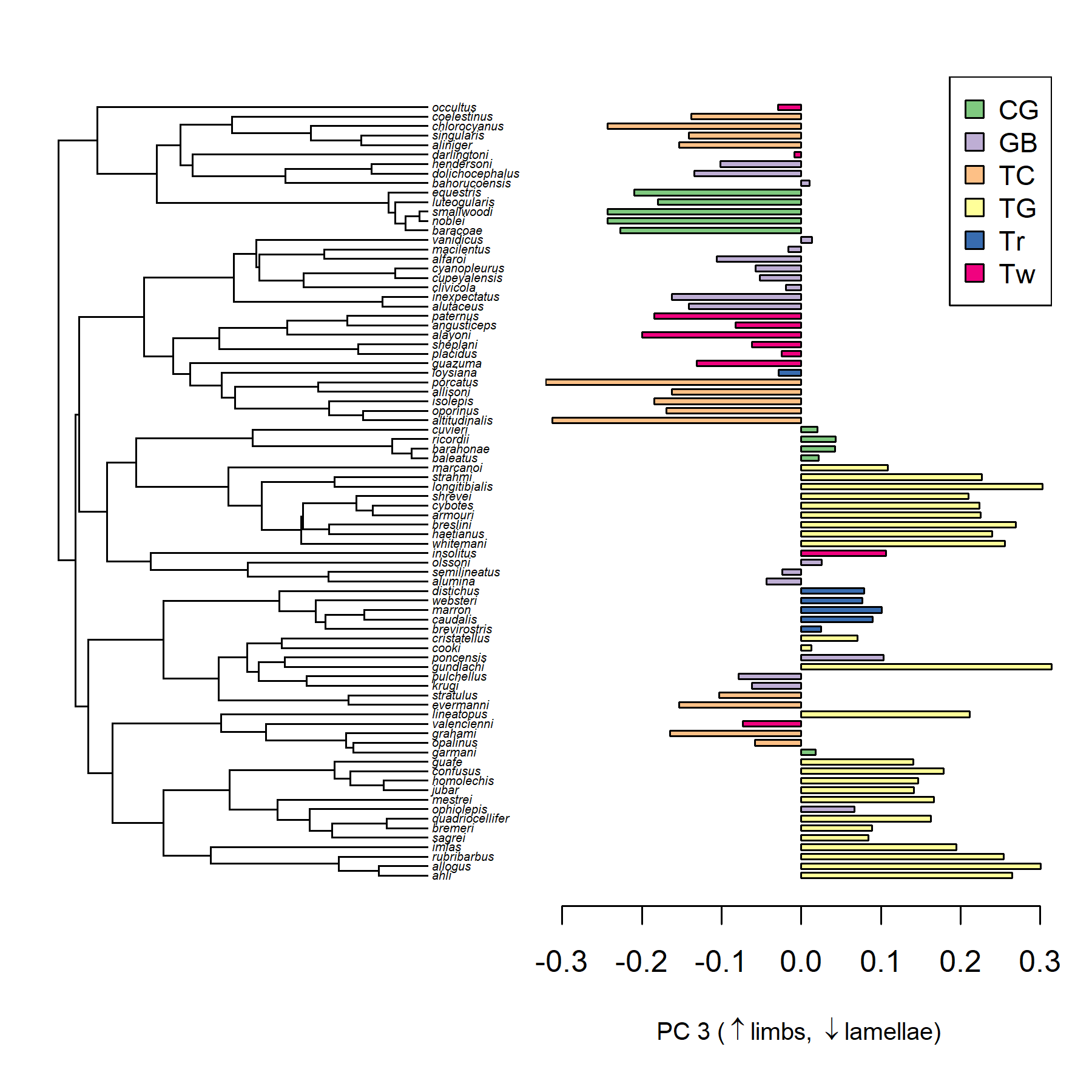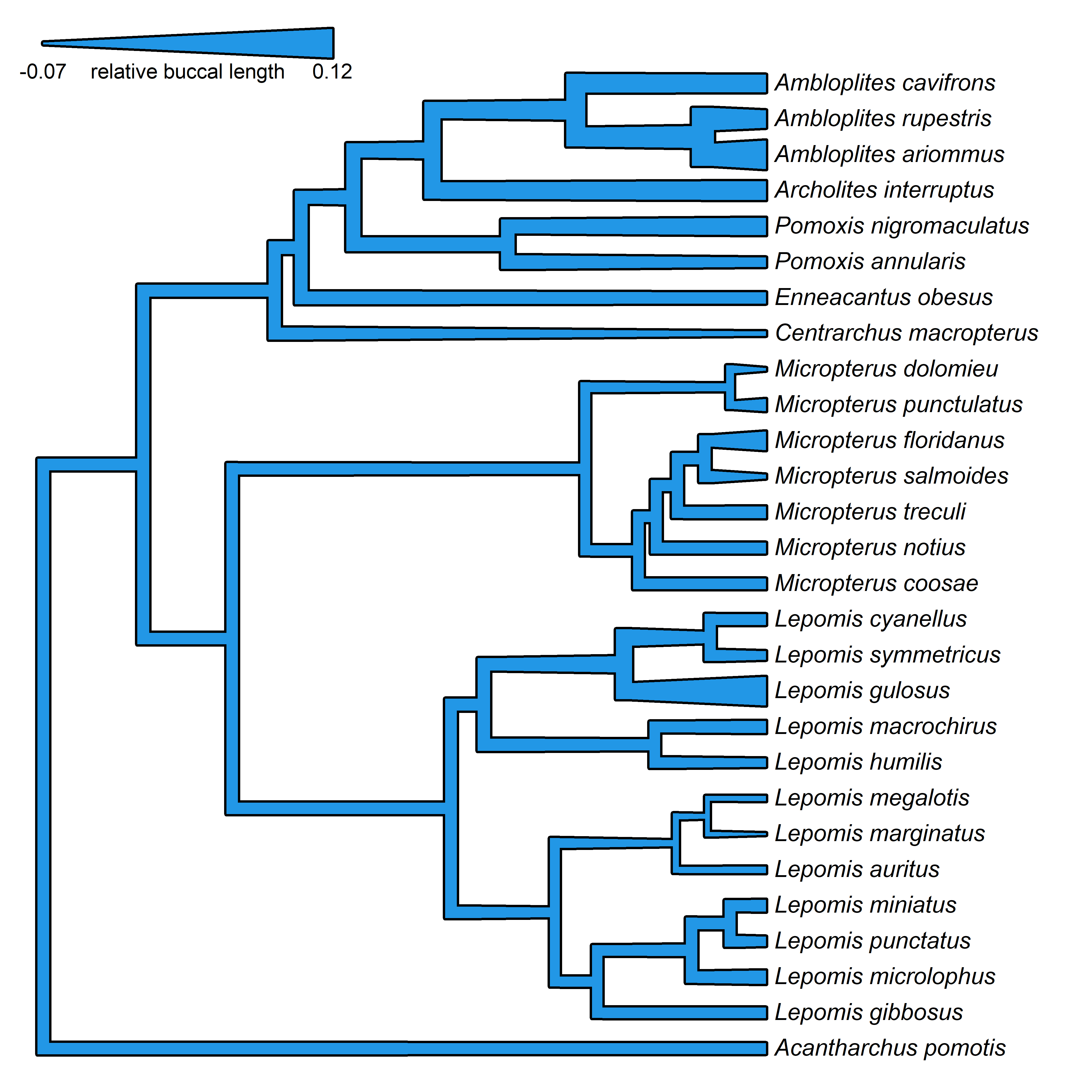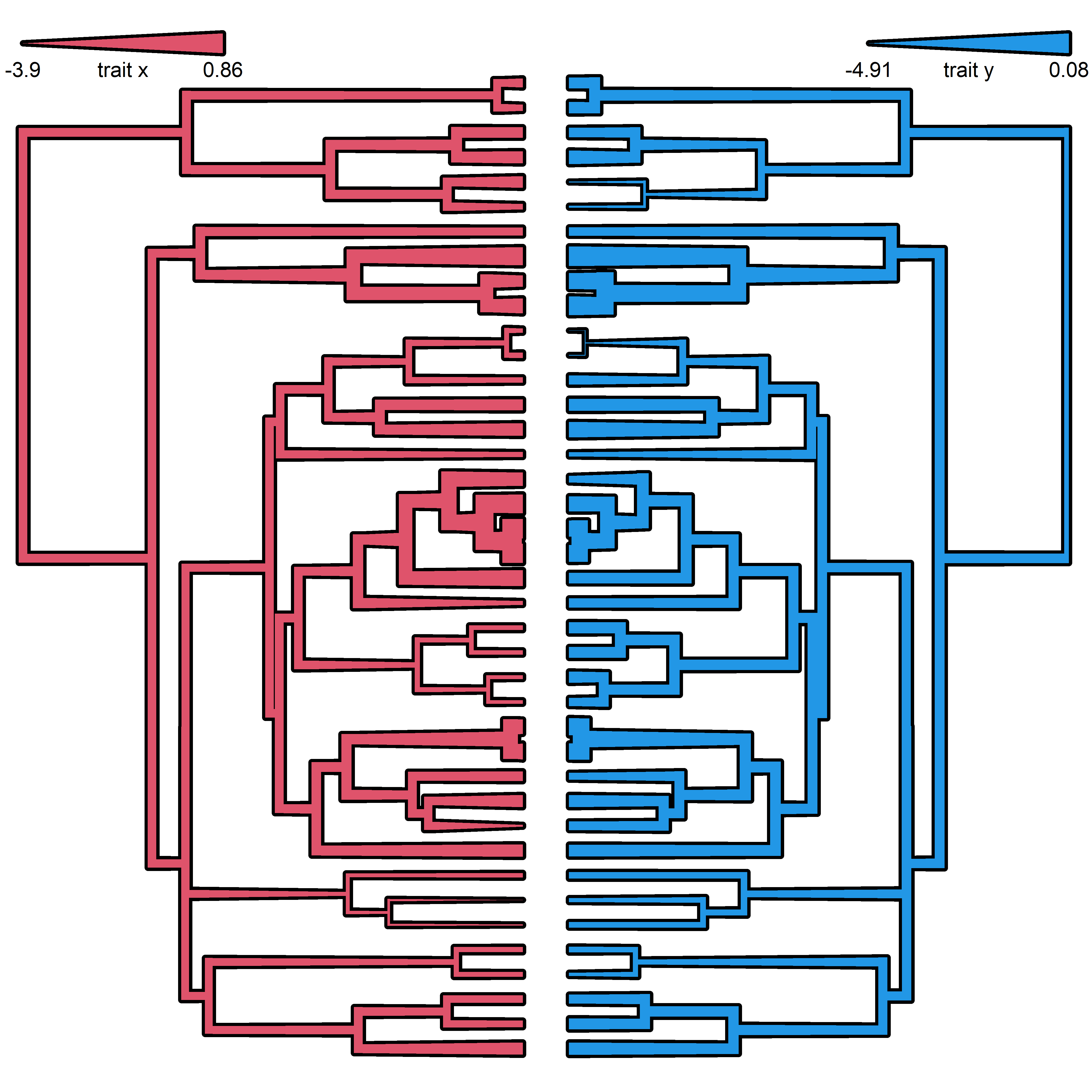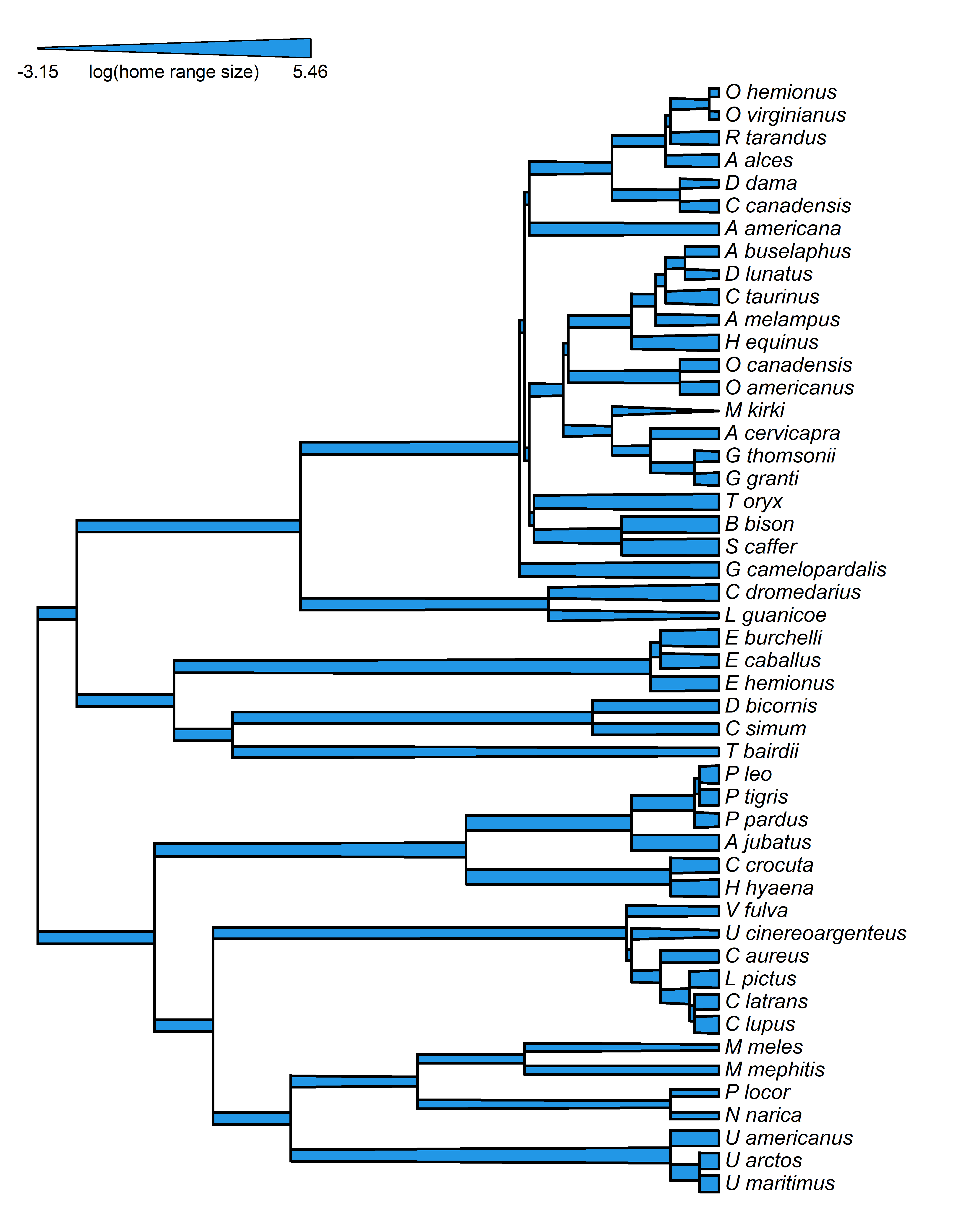## Friday, July 31, 2020

### Putting arrows (e.g., ↑,↓) into the axis labels of an R plot using `expression`

I was just working through the problem of trying to get up (↑) and down (↓) arrows into figure axis labels in R.

Just copying and pasting unicode arrows into the R script works for axis labels that are to be rendered by the R graphical device within an interactive R session.

Unfortunately, this does not work for figures or R markdown to be rendered as HTML (e.g., using knitr) or as PDFs (e.g., using rmarkdown)!

After digging online for some hints (the most useful one I found here, along with, of course, R help), the following is a better solution that uses the base function `expression`:

``````library(phytools)
tips<-getStates(ecomorph.tree,"tips")
tip.cols<-cols[tips]
plotTree.barplot(ecomorph.tree,scores(pca)[,3],
args.plotTree=list(fsize=0.4),
args.barplot=list(col=tip.cols,
xlab=expression(paste("PC 3 (",""%up%"","limbs, ",
""%down%"","lamellae)",sep="")),
cex.lab=0.8))
legend("topright",levels(anole.ecomorph[,1]),
pch=22,pt.bg=cols,pt.cex=1.5,cex=0.9)
``````Here are the tree & data that I used:

``````ecomorph.tree
``````
``````##
## Phylogenetic tree with 82 tips and 81 internal nodes.
##
## Tip labels:
##  ahli, allogus, rubribarbus, imias, sagrei, bremeri, ...
##
## The tree includes a mapped, 6-state discrete character with states:
##  CG, GB, TC, TG, Tr, Tw
##
## Rooted; includes branch lengths.
``````
``````pca
``````
``````## Phylogenetic pca
## Standard deviations:
##        PC1        PC2        PC3        PC4
## 0.81378367 0.22553447 0.12277042 0.10577197
##        PC5        PC6
## 0.04920179 0.03691686
##            PC1         PC2         PC3
## SVL -0.9712234  0.16067288  0.01972570
## HL  -0.9645111  0.16955087 -0.01203113
## HLL -0.9814164 -0.02674808  0.10315533
## FLL -0.9712265  0.17585694  0.10697935
## LAM -0.7810052  0.37429334 -0.47398703
## TL  -0.9014509 -0.42528918 -0.07614571
##             PC4         PC5         PC6
## SVL  0.14782215 -0.06211906  0.06935433
## HL   0.17994634  0.08064324 -0.04406887
## HLL -0.13790763  0.06887922  0.04126248
## FLL -0.09105747 -0.06075142 -0.04864769
## LAM -0.15871456  0.00217418  0.00875408
## TL   0.01709649 -0.01750404 -0.01088743
``````

That's all for now.

## Monday, July 20, 2020

### Mapping a continuous trait onto the branches of a phylogeny using variable edge widths: Part IV

A couple of days ago I posted some code showing how to plot a right- (or left-) facing phylogram with polygons for branches.

As of this afternoon, I have just pushed these new functions to the phytools GitHub page. This update can be obtained by downloading & installing phytools directly from GitHub using devtools.

``````library(devtools)
install_github("liamrevell/phytools")
``````

Beyond what I showed yesterday, the major feature that I added was the option to turn on borders around our polygons, and the option to plot the vertical lines connecting edges as simple lines (that is, without width).

Here's an example:

``````data(sunfish.tree)
data(sunfish.data)
buccal.length<-setNames(sunfish.data\$buccal.length,
rownames(sunfish.data))
bl.object<-edge.widthMap(sunfish.tree,buccal.length)
plot(bl.object,fsize=0.9,max.width=0.8,
legend="relative buccal length",
color=palette(),min.width=0.05,border="black",
lwd=3)
``````Alright, for fun let's simulate some data for two characters & apply it to facing trees:

``````tree<-pbtree(n=40)
X<-sim.corrs(tree,vcv=matrix(c(1,0.7,0.7,1),2,2))
par(mfrow=c(1,2))
plot(edge.widthMap(tree,X[,1]),color=palette(),
lwd=4,direction="rightwards",max.width=0.8,
ftype="off",legend="trait x",border="black")
plot(edge.widthMap(tree,X[,2]),color=palette(),
lwd=4,direction="leftwards",max.width=0.8,
ftype="off",legend="trait y",border="black")
``````Lastly, let's see what it looks like when we plot the vertical lines connecting edges in the plotted tree as simple lines (rather than as polygons) as was suggested in a response to my first post on this subject:

``````data(mammal.tree)
data(mammal.data)
lnHomeRange<-setNames(log(mammal.data\$homeRange),
rownames(mammal.data))
homeRange.fit<-edge.widthMap(mammal.tree,lnHomeRange)
homeRange.fit
``````
``````## Object of class "edge.widthMap" containing:
## (1) Phylogenetic tree with 49 tips and 48 internal nodes.
## (2) Vector of node values for a mapped quantitative
##     trait.
``````
``````plot(homeRange.fit,vertical.as.polygon=FALSE,
border="black",max.width=0.8,lwd=2,
color=palette(),legend="log(home range size)")
``````